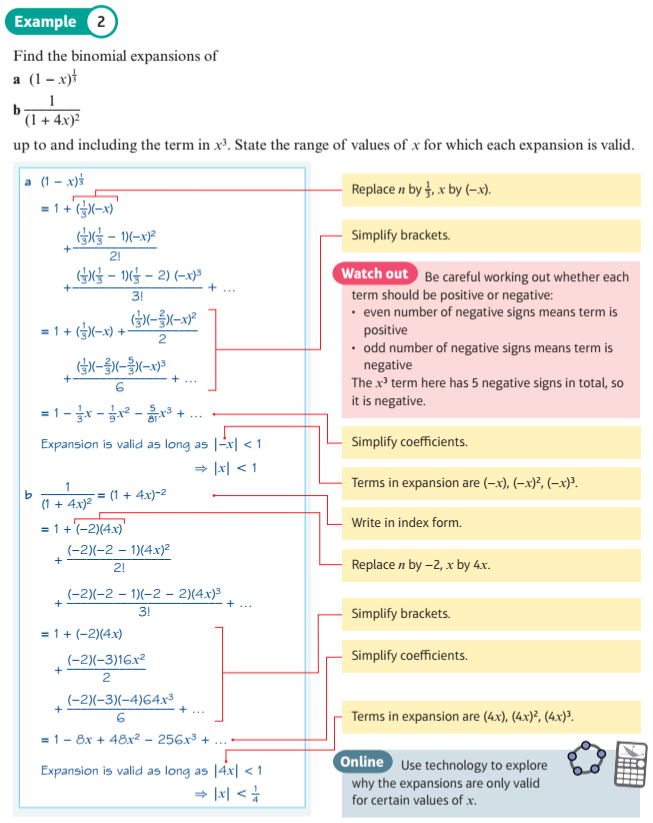Instant Maths Help:

# Edexcel A Level

## Binomial Expansion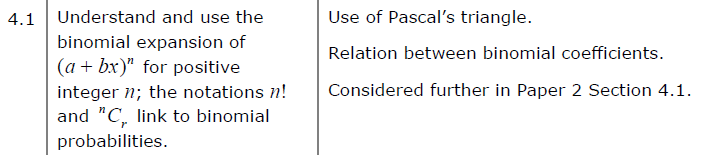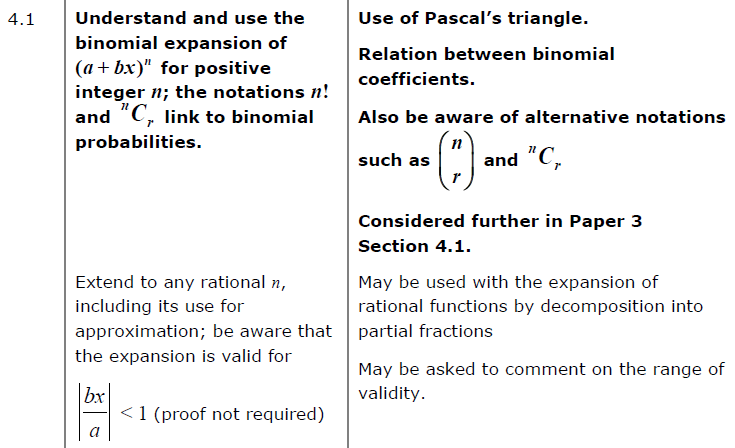##### Edexcel 9MA0/02 Jun 2023 A2 Exam Q. 13 :   13 marks in 15:36 min.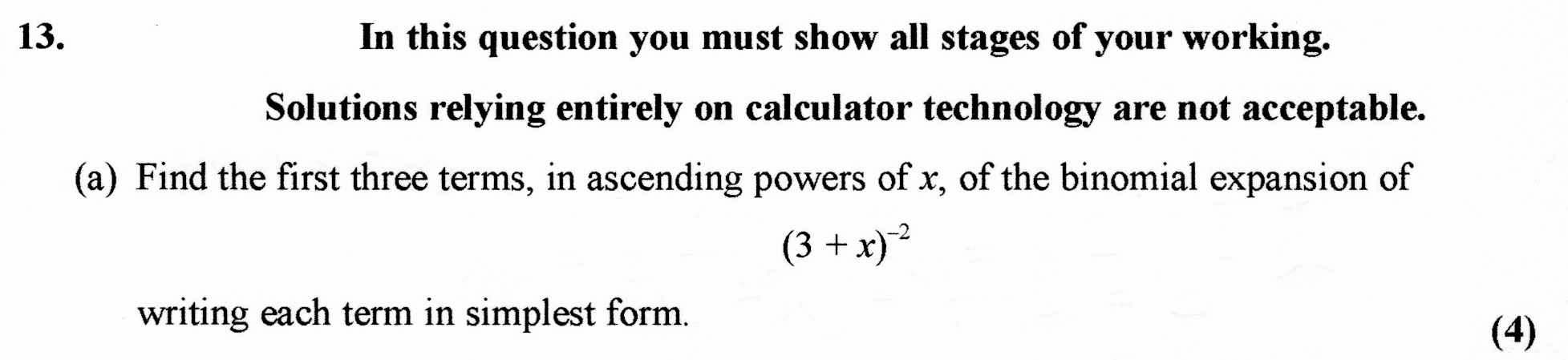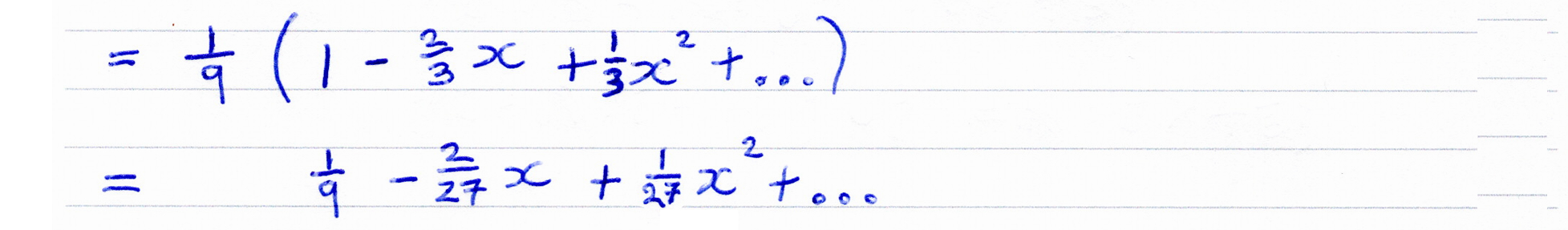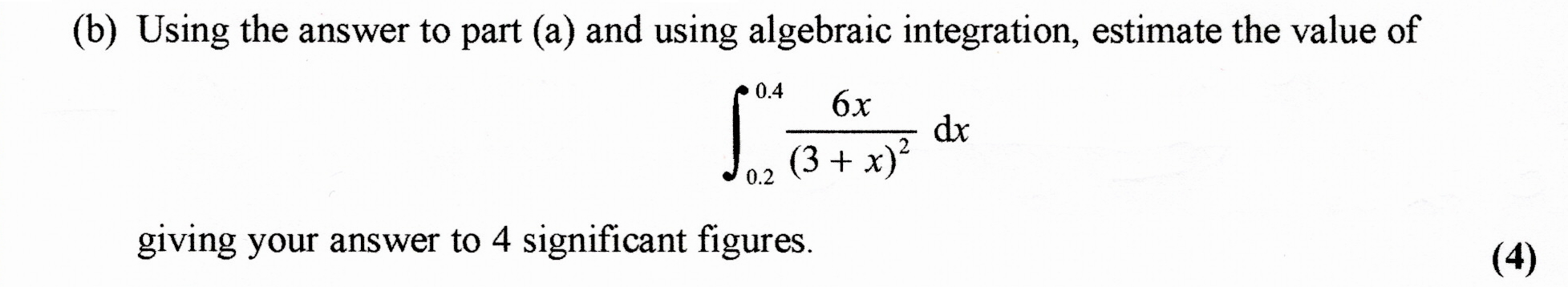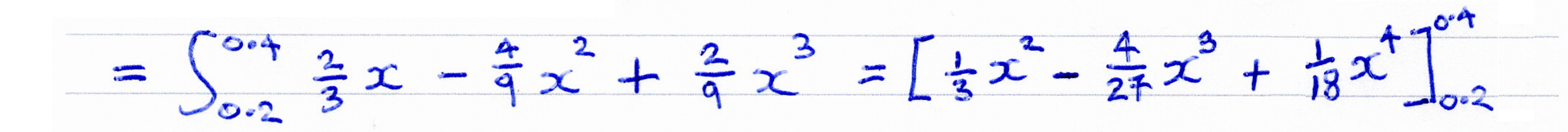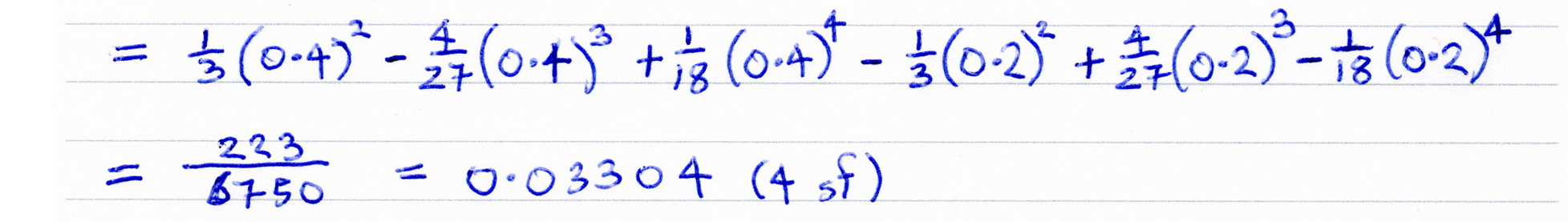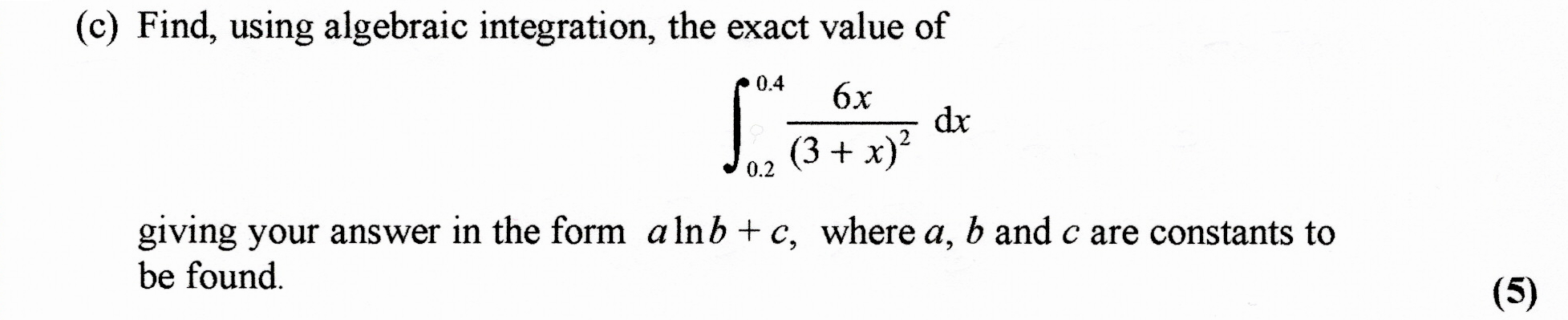##### Edexcel 8MA0/01 Jun 2023 AS Exam Q. 14 :   5 marks in 6:00 min.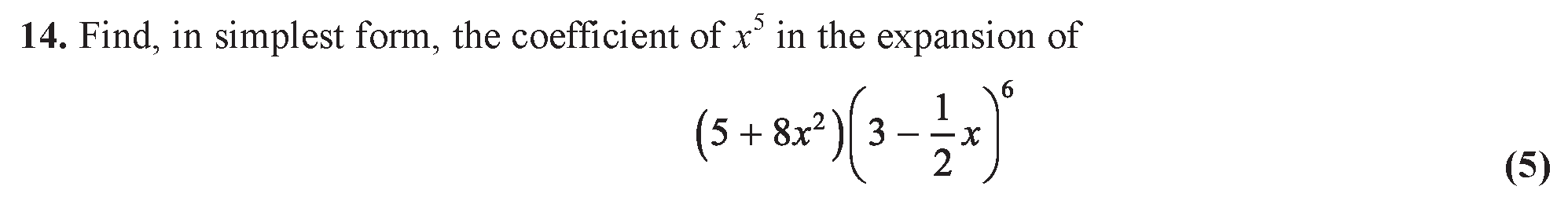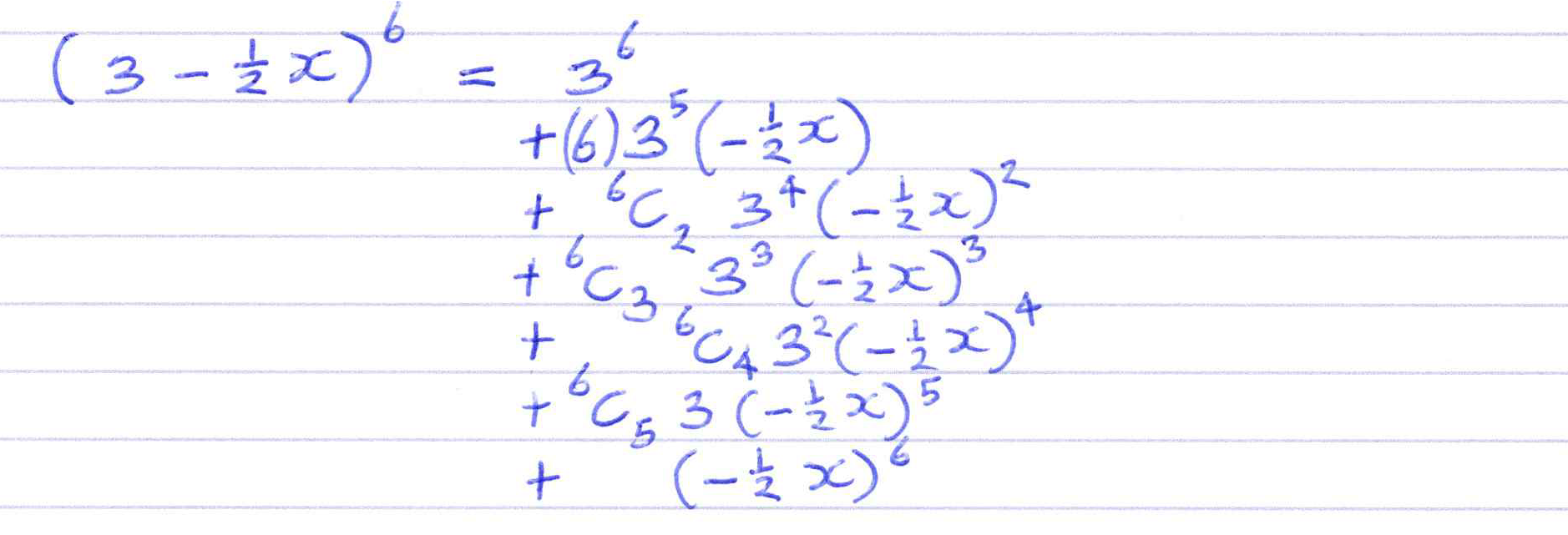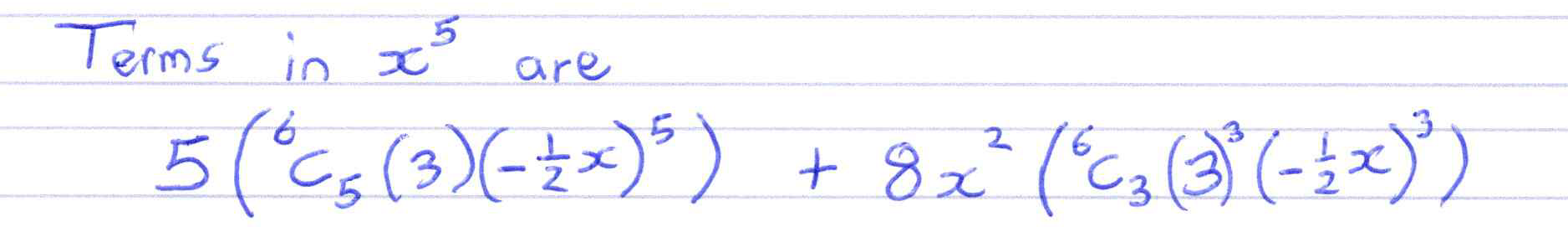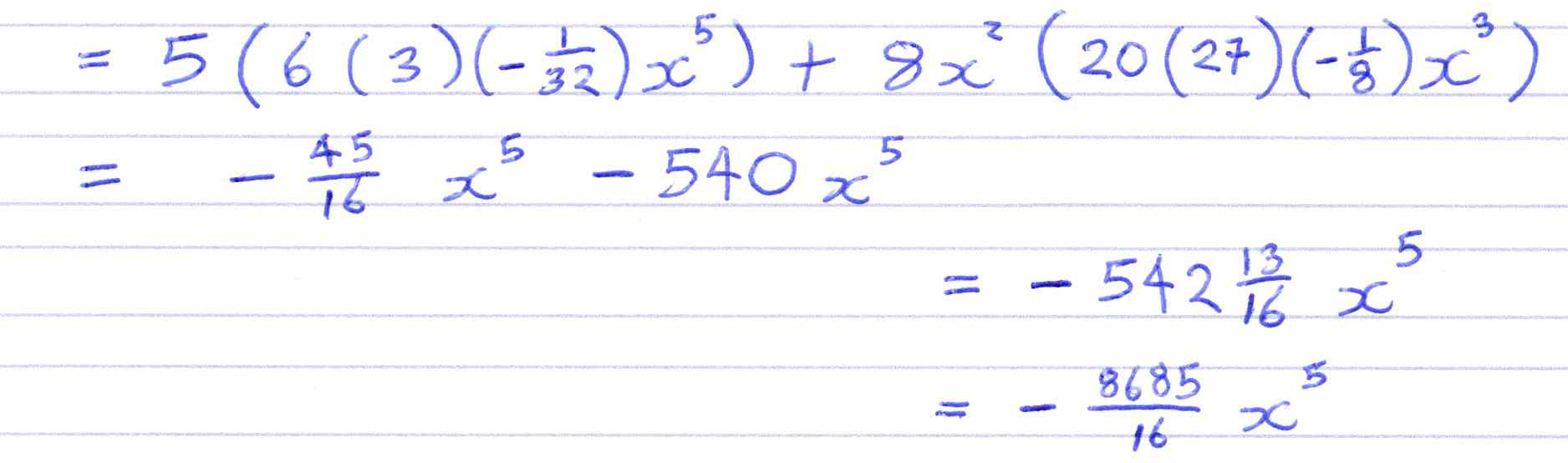##### Using combination and factorial functions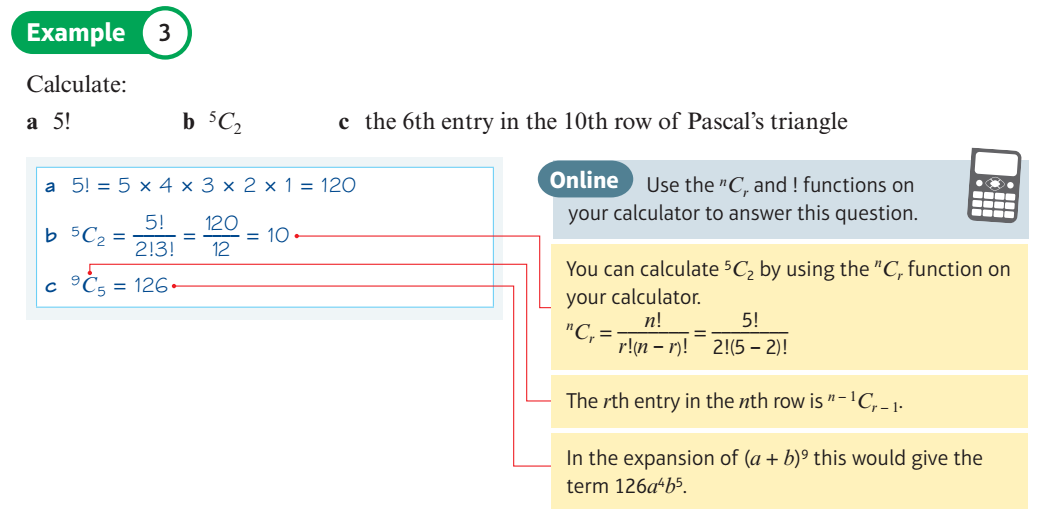##### Work out binomial coefficients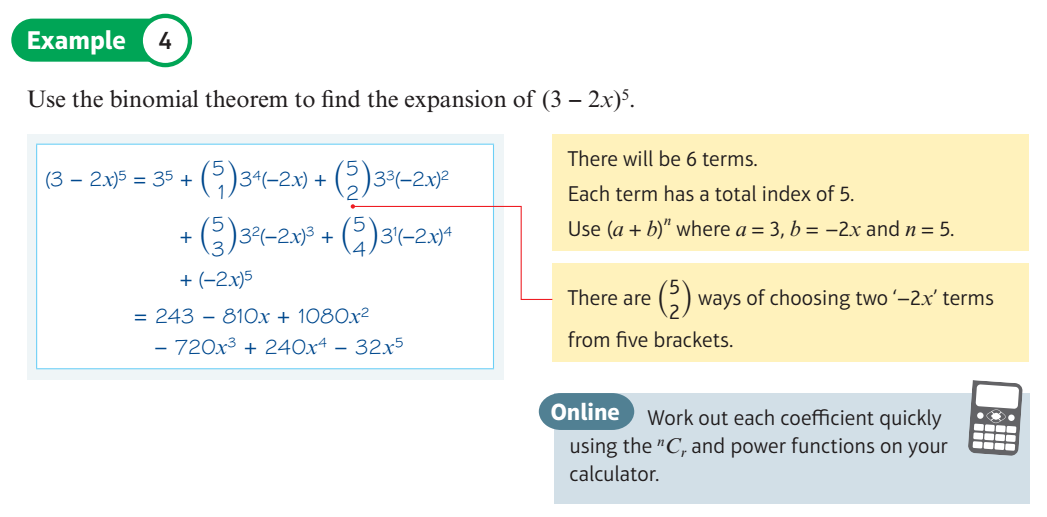##### Calculate coefficients for the binomial expansion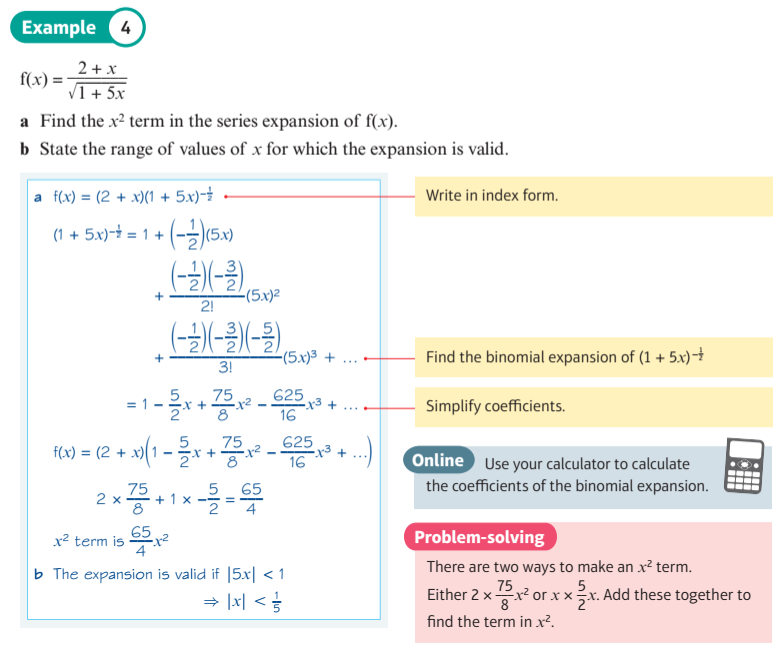##### Using a binomial expansion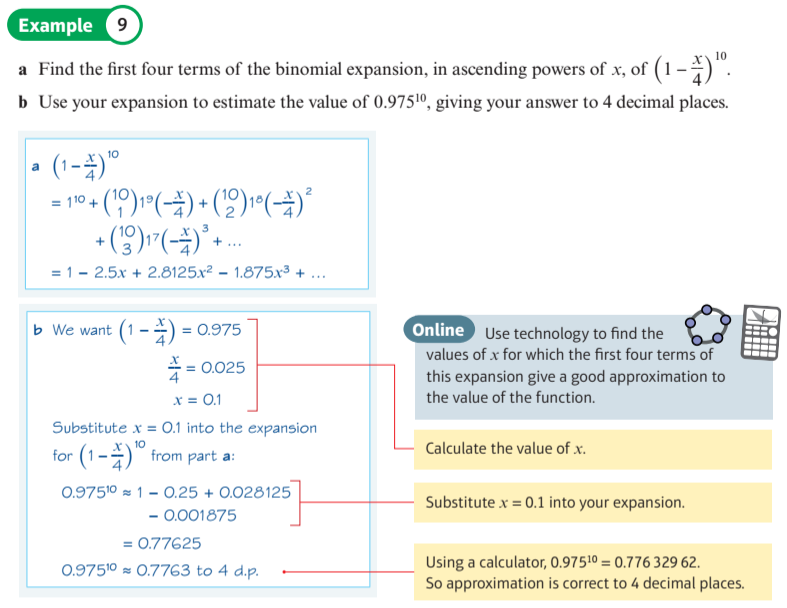##### Explore for which values of x the expansion is convergent, and therefore valid